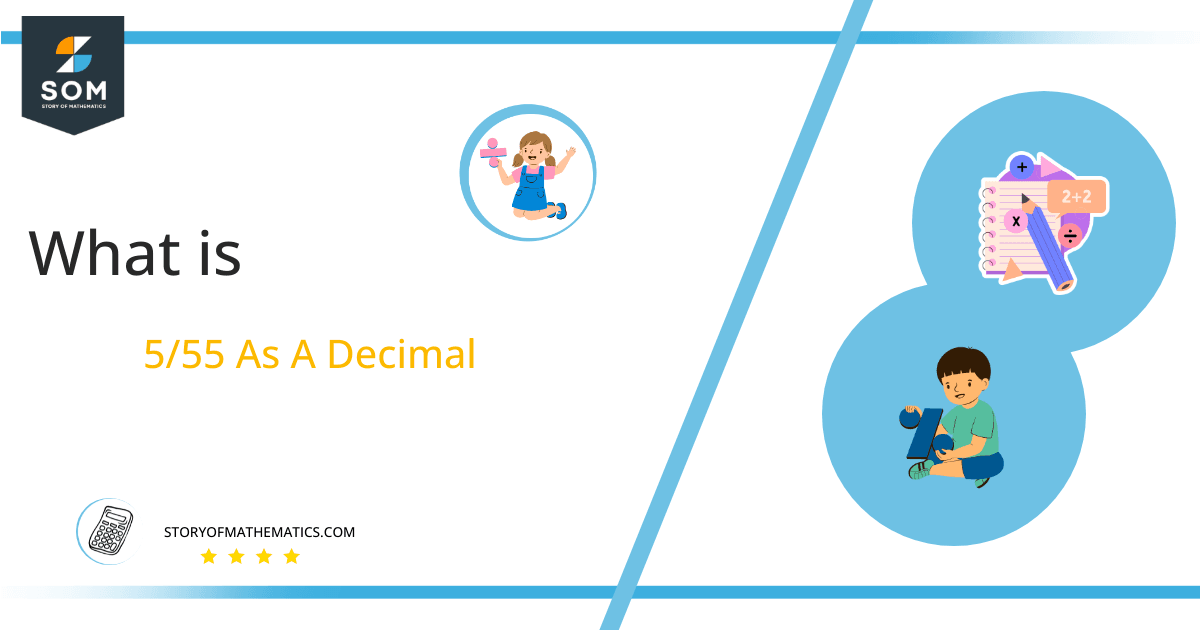# What Is 5/55 as a Decimal + Solution With Free Steps

The fraction 5/55 as a decimal is equal to 0.090.

The Fractions are transformed into decimal numbers when we divide both of their components. The Long Division method is an approach frequently used for this purpose. In this method, larger numbers are divided into smaller numbers of the same sizes or magnitudes.Here, we are more interested in the division types that result in a Decimal value, as this can be expressed as a Fraction. We see fractions as a way of showing two numbers having the operation of Division between them that result in a value that lies between two Integers.

Now, we introduce the method used to solve said fraction to decimal conversion, called Long Division, which we will discuss in detail moving forward. So, let’s go through the Solution of fraction 5/55.

## Solution

First, we convert the fraction components, i.e., the numerator and the denominator, and transform them into the division constituents, i.e., the Dividend and the Divisor, respectively.

This can be done as follows:

Dividend = 5

Divisor = 55

Now, we introduce the most important quantity in our division process: the Quotient. The value represents the Solution to our division and can be expressed as having the following relationship with the Division constituents:

Quotient = Dividend $\div$ Divisor = 5 $\div$ 55

This is when we go through the Long Division solution to our problem, as shown in figure 1.## 5/55 Long Division Method

We start solving a problem using the Long Division Method by first taking apart the division’s components and comparing them. As we have 5 and 55, we can see how 5 is Smaller than 55, and to solve this division, we require that 5 be Bigger than 55.

This is done by multiplying the dividend by 10 and checking whether it is bigger than the divisor or not. If so, we calculate the Multiple of the divisor closest to the dividend and subtract it from the Dividend. This produces the Remainder, which we then use as the dividend later.

Now, we begin solving for our dividend 5, which after getting multiplied by 10 becomes 50.

We take this 50 and divide it by 55; this can be done as follows:

50 $\div$ 55 $\approx$ 0

Where:

55 x 0 = 0

This will lead to the generation of a Remainder equal to 50 – 0 = 50. Now this means we have to repeat the process by Converting the 50 into 500 and solving for that:

500 $\div$ 55 $\approx$ 9

Where:

55 x 9 = 495

This, therefore, produces another Remainder which is equal to 500 – 495 = 5. Now we must solve this problem to Third Decimal Place for accuracy, so we repeat the process with dividend 50.

50 $\div$ 55 $\approx$ 0

Where:

55 x 0 = 0

Finally, we have a Quotient generated after combining the three pieces of it as 0.090=z, with a Remainder equal to 50.Images/mathematical drawings are created with GeoGebra.